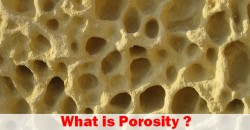## What is Porosity?Porosity is one of rock important rock properties and it is a measure of pore space in a rock.Figure 1 – Porosity

(Ref: http://www.tulane.edu/~sanelson/images/pores.gif)

Figure 1 shows the illustration of porosity and it can be seen that grain size and distribution can affect porosity of rock.

Porosity (ɸ) is mathematically expressed as a ratio of total void space with a rock to a total volume of rock.

## Porosity (ɸ) = (Vp ÷ Vb) × 100

Where;

Vp = pore volume

Vb = bulk volume of rock

Porosity (ɸ) is shown in percentage form. Continue reading